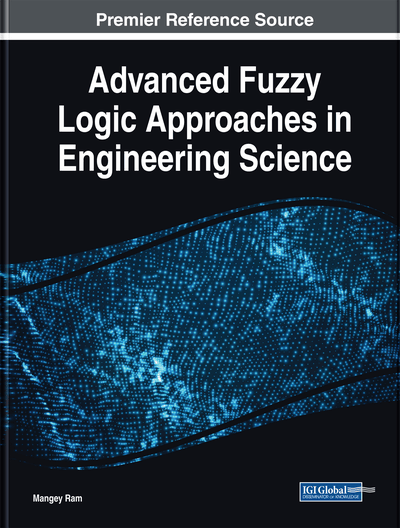# Multi-Criteria Decision-Making Approach Based on Moderator Intuitionistic Fuzzy Hybrid Aggregation Operators

Bhagawati Prasad Joshi (Seemant Institute of Technology, India) and Akhilesh Singh (Seemant Institute of Technology, India)
DOI: 10.4018/978-1-5225-5709-8.ch011
Available
\$37.50
No Current Special Offers

## Abstract

It has been seen in literature that the notion of intuitionistic fuzzy sets (IFSs) is very powerful tool to deal with real life problems under the environment of uncertainty. This notion of IFSs favours the intermingling of the uncertainty index in membership functions. The uncertainty index is basically generated from a lot of parameters such as lack of awareness, historical information, situation, short of standard terminologies, etc. Hence, the uncertainty index appended finding the membership grade under IFSs needs additional enhancement. Then, the concept of a moderator intuitionistic fuzzy set (MIFS) is defined by adding a parameter in the IFSs environment to make the uncertain behaviour more accurate. In this chapter, some new moderator intuitionistic fuzzy hybrid aggregation operators are presented on the basis of averaging and geometric point of views to aggregate moderator intuitionistic fuzzy information. Then, a multi-criteria decision-making (MCDM) approach is provided and successfully implemented to real-life problems of candidate selection.
Chapter Preview
Top

## Introduction

The concept of fuzzy sets (FSs) was introduced by Zadeh (1965) as the extension of traditional classical sets. Further, Atanassov (1986) extended FSs by introducing the concept of intuitionistic fuzzy sets (IFSs). This extension of FSs is more powerful technique to deal with vagueness in real life problems and achieved much more attention to researchers. IFSs theory is widely applicable in decision-making, forecasting problems, medical diagnosis, data analysis, artificial intelligence, etc. Joshi and Kumar (2012a, 2012b, 2013) successfully applied the concept of IFSs in forecasting problems. Atanassov (1989, 1994), De et al. (2000), Xu (2005, 2007), Xu and Da (2002) and Xu and Yager (2006) did pioneering standard research on the intuitionistic fuzzy operational laws and the development of aggregation operators. Atanassov (1994) proposed addition and multiplication operation and De et al. (2000) defined scalar multiplication operation and power operation over intuitionistic fuzzy numbers (IFNs). Based on these laws and operations, Xu (2007) introduced some intuitionistic fuzzy aggregation operators such as: intuitionistic fuzzy weighted averaging (IFWA) operator, intuitionistic fuzzy ordered weighted averaging (IFOWA) operator and intuitionistic fuzzy hybrid averaging (IFHA) operator. Xu and Yager (2006) developed some intuitionistic fuzzy geometric aggregation operators such as: intuitionistic fuzzy weighted geometric averaging (IFWGA) operator, intuitionistic fuzzy ordered weighted geometric averaging (IFOWGA) operator and intuitionistic fuzzy hybrid geometric averaging (IFHGA) operator. Wang and Liu (2011) developed some new geometric aggregation operators, such as the intuitionistic fuzzy Einstein weighted geometric operator and the intuitionistic fuzzy Einstein ordered weighted geometric operator based on Einstein sum, Einstein product and Einstein exponentiation. Wang and Liu (2012) presented intuitionistic fuzzy Einstein weighted averaging operator and the intuitionistic fuzzy Einstein ordered weighted averaging operator, which extend the weighted averaging operator and the ordered weighted averaging operator to aggregate intuitionistic fuzzy values. Zhao and Wei (2013) developed some new Einstein hybrid aggregation operators, such as the intuitionistic fuzzy Einstein hybrid averaging operator and intuitionistic fuzzy Einstein hybrid geometric operator. After these interesting researches, aggregation operators have attracted much attention from researchers (Tan & Chen, 2010; Zhao, Xu, Ni, & Liu, 2010; Ye, 2010; Beliakov, Bustince, Goswami, Mukherjee, & Pal, 2011; Xu & Xia, 2011; Xu, 2011; Xu & Yager, 2011; Zhou & Chen, 2012; Xia, Xu, & Zhu, 2012; Xu & Wang, 2012; Yu & Xu, 2013; Zhang, 2013; He, Chen, Zhou, Liu, & Tao, 2014; Lion & Xu, 2014; Joshi, 2016a; Joshi, 2016b; Joshi & Kumar, 2016; Joshi, 2017)

## Complete Chapter List

Search this Book:
Reset